# Modeling Electrolytically Top-Gated Graphene

## Abstract

We investigate doping of a single-layer graphene in the presence of electrolytic top gating. The interfacial phenomenon is modeled using a modified Poisson–Boltzmann equation for an aqueous solution of simple salt. We demonstrate both the sensitivity of graphene’s doping levels to the salt concentration and the importance of quantum capacitance that arises due to the smallness of the Debye screening length in the electrolyte.

## Introduction

Carbon nano-structures show great promise in many applications, including chemical and biological sensors. While carbon nanotubes (CNTs) have been extensively studied in that context for quite some time [1, 2], investigations of graphene as a sensor are only beginning to appear . Sensory function of carbon nano-structures is generally implemented in the configuration of a field effect transistor (FET), with a prominent role played by the gate potential that controls the current through the device. Biochemical applications require good understanding of the interaction of carbon nano-structures with aqueous solutions , often in the context of the electrochemical top gating . While significant progress has been achieved in understanding the interaction of CNT–FETs with the electrolytic environment , similar studies involving graphene have appeared only very recently , focusing on the screening effect of an ion solution on charge transport through graphene-based FETs , as well as on the measurement of the quantum capacitance of graphene as an ultimately thin electrode in an aqueous solution .

The top gating of a graphene-based FET with a solid or liquid electrolyte presents several advantages compared to the conventional back gating with a metallic electrode. Upon application of gate voltage, free ions in the electrolyte re-distribute themselves, forming an electrostatic double layer (EDL) at the interface between graphene and the electrolytic solution . Depending on the ion concentration, the EDL can be only a few nanometers thick, while still providing efficient shielding of graphene. As a consequence, the capacitance of the EDL in an electrolyte can be much higher than the capacitance of the back gate, which is typically separated from graphene with a layer of SiO2 a few hundred nanometers thick . This property of the EDL enables a much better control of the surface potential on the graphene layer, while requiring a much lower operating voltage that needs to be applied to the reference electrode in the electrolyte than voltages currently used with back gates . The applied voltage then modifies the chemical potential of graphene, resulting in changes in its observable properties such as conductance. Since properties of the EDL depend on the ion concentration, monitoring the resulting changes in graphene’s conductance can provide a means for sensor application, e.g., in measuring the amount of salt in the solution.

On the other hand, referring to the electrical model of the electrolytic gating as a series connection of capacitors , the high gate capacitance in the electrolyte gives a much more prominent role to the quantum capacitance of graphene than does the back gate [11, 1416]. In addition, doping levels of an electrolytically top-gated graphene have been reported recently  to be much higher than those obtained with the conventional back gate . At the same time, mobile ions in the solution seem to provide a much more effective screening of charged impurities underneath the graphene, thereby significantly increasing the charge carrier mobility in graphene in comparison with some other high-κ dielectric environments . All these facts indicate that electrolytic top gating provides a means to develop high-performance FETs.

While the above few experimental observations reveal quite fascinating aspects of the graphene–electrolyte interaction, theoretical modeling of this system seems to be lagging behind the experiment. It is therefore desirable and tempting to discuss doping of a single layer of graphene by a remote gate electrode immersed in a thick layer of electrolyte by using two simple models: one describing graphene’s π electron band structure in the linear energy dispersion approximation , and the other describing the distribution of ions in the electrolyte by a one-dimensional (1D) Poisson–Boltzmann (PB) model, which takes advantage of the planar symmetry of the problem . However, it should be emphasized that the experiments involving electrolytic top gating of both CNTs [7, 8] and graphene [6, 10] use rather high voltages, on the order of 1–2 V, which can cause significant crowding of counter-ions at the electrolyte–graphene interface. It is therefore necessary to go beyond the standard PB model by taking into account the steric effects, i.e., the effects of finite size of ions in the solution. To that aim, we shall use the modified Poisson–Boltzmann (mPB) model developed by Borukhov et al. [22, 23], which retains analytical tractability of the original 1D-PB model. In addition, applied voltages beyond 1 V also require taking into account non-linearity of graphene’s band energy dispersion, giving small but noticeable corrections to the linear approximation.

We shall consider here a simple 1:1 electrolyte representing an aqueous solution of NaF because both the Na+ and the F ions are chemically inert allowing us to neglect their specific adsorption on the graphene surface [10, 13]. In particular, we shall analyze the density of doped charge carriers in graphene at room temperature (RT) as a function of both the applied voltage and the salt concentration to elucidate graphene’s sensor ability. In addition, we shall evaluate the contributions of both the graphene and the EDL in the total gate capacitance in terms of the applied voltage to reveal the significance of quantum capacitance, as well as to elucidate the behavior of the EDL under high voltages. We shall cover broad ranges of both the salt concentration, going from μM to a physiologically relevant value, and the applied voltage, going up to about 2 V.

After outlining our theoretical models for graphene and the EDL in the next section, we shall introduce several reduced quantities of relevance for these two vastly different systems and present our results in the following section. Finally, conclusion follows. Note that we shall use gaussian units (4πε0 = 1) throughout the paper, unless otherwise explicitly stated.

## Theoretical Model

Graphene is a semi-metal, or a zero-gap semiconductor because its conducting and valence π electron bands touch each other only at two isolated points in its two-dimensional (2D) Brillouin zone . The conical shape of these bands in the vicinity of these points gives rise to an approximately linear density of states,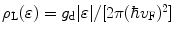, where gd = 4 is the spin and the band valley degeneracy factor, and vFc/300 is the Fermi speed of graphene, with c being the speed of light in vacuum . In the intrinsic, or undoped graphene, the Fermi energy level sits precisely at the neutrality point, ɛF = 0, also called the Dirac point. Therefore, the electrical conductivity of graphene is easily controlled, e.g., by applying a gate voltage VA that will cause doping of graphene’s π bands with electrons or holes (depending on the sign of VA), which can attain the number density per unit area, n, with a typical range of n 1011–1013 cm−2. In a doped graphene, Fermi level moves to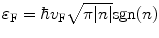, where sgn(n) = ± 1 for electron (hole) doping. At a finite temperature T, one can express the charge carrier density in a doped graphene in terms of its chemical potential μ as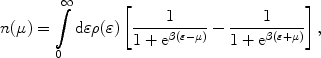(1)

where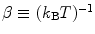with kB being the Boltzmann constant. We shall use in our calculations a full, non-linear expression for the π electron band density, ρ(ɛ), given in Eq. 14 of Ref. . However, for the sake of transparency, the theoretical model for graphene will be outlined below within the linear density approximation, ρ(ɛ) ≈ ρL (ɛ). We note that this approximation is accurate enough for low to moderate doping levels, such that, e.g.,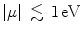, and it only incurs a relative error of up to a few percent when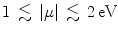.

At this point, it is convenient to define the potential VQ = −μ/e, where e > 0 is the proton charge, which is associated with the quantum-mechanical effects of graphene’s band structure , and relate it to the induced charge density per unit area on doped graphene, σ = − en, via the Eq. 1,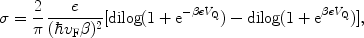(2)

where dilog is the standard dilogarithm function . One can finally use the definition of differential capacitance per unit area, CQ = dσ/dVQ, to obtain from Eq. 2 the quantum capacitance of a single layer of graphene as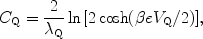(3)

where we have defined the characteristic length scale for graphene,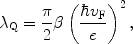(4)

with the value of λQ ≈ 18 nm at RT. Note from Eq. 3 that graphene’s quantum capacitance grows practically linearly with VQ when this potential exceeds the thermal potential, Vth = 1/(e β), having the value of ≈26 mV at RT.

We further assume that an upper surface of graphene is exposed to a thick layer of a symmetric z: z electrolyte containing the bulk number density per unit volume, N, of dissolved salt ions. Taking advantage of planar symmetry, we place an x axis perpendicular to graphene and pointing into the electrolyte. The theory developed by Borukhov et al. [22, 23] to model finite ion size uses the mPB equation for the electrostatic potential V (x) in the electrolyte at a distance x from graphene, given by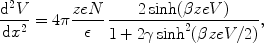(5)

where z(=1) is the valency of ions, ε is relative dielectric constant of water (≈80, assumed to be constant throughout the electrolyte), and γ = 2a3N is the packing parameter of the solvated ions, which are assumed to have same effective size, equal to a[22, 23]. We note that the standard PB model is recovered from Eq. 5 in the limit γ → 0 . By assuming the boundary condition V(x) = 0 (and hence dV/dx = 0) at x → ∞, deep into the electrolyte bulk, Eq. 5 can be integrated once giving a relation between the electric field and the potential at a distance x from graphene. Assuming that graphene is placed at x = 0, one can use the boundary condition at the distance d of closest approach for ions in the electrolyte to graphene,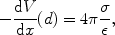(6)

to establish a connection between the induced charge density on graphene, σ, and the potential drop, VD = V(d), across the EDL as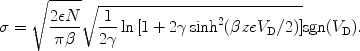(7)

The total potential, VA, applied between the reference electrode in the electrolyte and graphene can be written as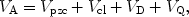(8)

where Vpzc = (WgrWref)/e is the potential of zero charge  that stems from difference between the work functions of graphene and the reference electrode, Wgr and Wref, respectively, and Vcl = 4πhσ/ε′ is the potential drop across a charge-free region between the compact layer of the electrolyte ions condensed on the graphene surface, having the thickness h on the order of the distance of closest approach d[23, 24], and with ε′ < ε taking into account a reduction of the dielectric constant of water close to a charged wall . In our calculations, we shall neglect these two contributions to the applied potential in Eq. 8 because Vpzc merely shifts the zero of that potential, while a proper modeling of Vcl involves large uncertainty [23, 24]. However, usually the effects of Vcl can be considered either small  or incorporated in the mPB model via saturation of the ion density at the electrolyte–graphene interface for high potential values . Consequently, VD and VQ represent the two main contributions in Eq. 8, with VD being the surface potential of graphene that shifts its Dirac point, and VQ being responsible for controlling the doping of graphene by changing its chemical potential. Finally, we note that all results of our calculations will be symmetrical relative to the change in sign of the applied potential because of our assumption that the effective sizes of the positive and negative ions are equal [22, 23], but this constraint can be lifted by a relatively simple amendment to the mPB model .

Using the relation VA = VQ + VD, we obtain the total differential capacitance of the electrolytically top-gated graphene as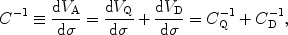(9)

where CQ(VQ) is given in Eq. 3, and CD(VD) = dσ/dVD is the differential capacitance per unit area of the EDL, which can be obtained from Eq. 7as [23, 24],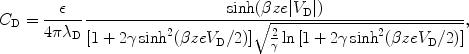(10)

withbeing the Debye length of the EDL . Note that, in the limit of a very low potential VD, and hence for low density of ions at the graphene–EDL interface, one can set γ → 0 in Eq. 10 to recover an expression for the EDL capacitance in the standard PB model ,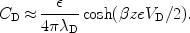(11)

We further note that, while Eq. 11 implies an unbounded growth of the EDL capacitance with VD in the PB model, Eq. 10 suggests a non-monotonous behavior that will eventually give rise to a saturation of the total gate capacitance at high applied voltages.

## Results

Given the vast ranges of various parameters of interest in our model, it is of interest to define reduced quantities. With the thermal potential Vth = 1/(e β), all potentials can be written as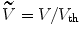. While typical regimes of graphene doping require only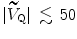, we shall extend this range in our calculations up to about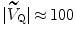to represent doping levels in recent experiments in electrolytic environment . Next, referring to Eq. 2, we define the characteristic number density of doped charge carriers in graphene by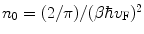, which has the value of n0 ≈ 1011 cm−2 at RT. Therefore, defining the reduced density by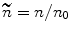, and hence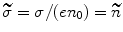, we note that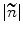may reach up to around 103[6, 12]. It is worthwhile mentioning that graphene’s characteristic parameters λQ and n0 are related via ελBλQn0 = 1, where λB = βe2/ε is the Bjerrum length of the aqueous environment, taking the value of λB ≈ 0.7 nm at RT . Furthermore, it follows from Eq. 3 that the natural unit of capacitance for this system is C0 = en0/Vth = λQ−1, taking the value of C0 ≈ 0.6 μF/cm2 at RT. Turning now to Eq. 7, one can define the characteristic number density of ions per unit volume in the solution by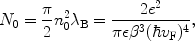(12)

which takes the value of N0 ≈ 1.08 × 10−6 nm−3 ≈ 1.8 μM at RT. Defining the reduced concentration of ions in the bulk of the electrolyte by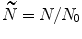, it would be of interest to explore a broad range of its values, e.g.,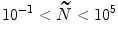. Finally, in order to estimate the packing parameter, we write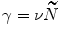and take a = λB to obtain ν = 2λB3N0 ≈ 7 × 10−7. With these definitions, Eqs. 2 and 7 now read, respectively,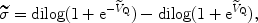(13)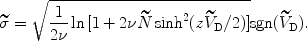(14)

We now use Eqs. 13and14in conjunction with the relation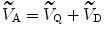to eliminate the potential components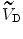and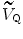, and to evaluate the reduced density of doped charge carriers in graphene,, as a function of the reduced applied voltage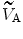and the reduced salt concentration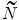. The results are shown in Figs. 1 and 2, covering the following ranges: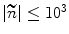(corresponding to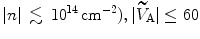(corresponding to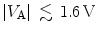), and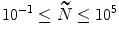(corresponding to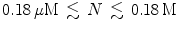). In Fig. 1, one notices a strong dependence of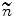on the applied potential forgreater than about 30, which gives approximately equal rates of change for each salt concentration at the highest values of the applied potential. On the other hand, at the lower applied potential values, there exists a much stronger dependence on the salt concentration, which is revealed in Fig. 2, showing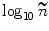versus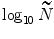for several applied voltages. Indeed, one notices a very strong sensitivity of the doped charge carrier density in graphene to the salt concentration for applied voltages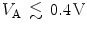in the range of salt concentrations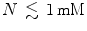. Even though this sensitivity seems to be the strongest at the lowest applied voltages, one should bear in mind that the electrical conductivity in graphene becomes rather uncertain around its minimum value, which extends up to doping densities about n ≈ 1011 cm−2[26, 27]. Therefore, it seems that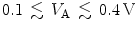would be an optimal range of applied voltages for sensor applications of the electrolytically top-gated graphene in probing salt concentrations in the sub-millimole range.

Next, moving to the capacitance of electrolytically top-gated graphene, C, we note that the reduced capacitances,and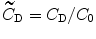, are obtained from Eqs. 3and10as(15)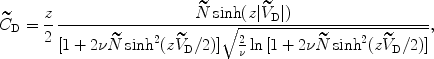(16)

showing that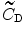and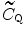are comparable in magnitude for vanishing potentials when the salt concentration is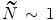. Moreover, referring to Eq. 9as an electrical model where graphene and the EDL act as a series connection of capacitors, it follows that graphene’s quantum capacitance CQ will be promoted as the dominant contribution to the total gate capacitance as the salt concentration increases.

We now use the equality of the right-hand-sides in Eqs. 13and14along with the relationto eliminateandand to evaluate the reduced quantum capacitance of graphene from Eq. 15, as well as the reduced capacitance of the EDL from Eq. 16as functions of the reduced applied voltage. Results are shown in Fig. 3 along with the total reduced capacitance of the system based on Eq. 9, for two reduced salt concentrations,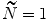= 1 and 105(corresponding to N ≈ 1.8 μM and 0.18 M, respectively). We show our results for the reduced applied voltages up to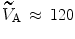in order to elucidate the effect of saturation in the total capacitance that occurs at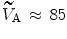(corresponding to VA ≈ 2.21 V) forand at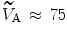(corresponding to VA ≈ 1.95 V) for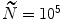. As can be seen from dotted curves in Fig. 3, showing a non-monotonous dependence of the EDL capacitance on the applied voltage, the saturation effect in the total capacitance of the electrolytically top-gated graphene is a consequence of the steric effect of the electrolyte ions that are crowded at the graphene surface at high applied voltages . Even though the voltages where the saturation occurs are relatively high, they may still be accessible in experiments on graphene. Furthermore, we see in Fig. 3 that at intermediate applied voltages, the rate of change of the total capacitance follows closely that of the quantum capacitance, with the value ≈23 μF/(V cm2) that is commensurate with recent measurement . At the lowest applied voltages, one notices in Fig. 3 a “rounding” of the total capacitance as a function of voltage for low salt concentrations, which comes from the EDL capacitance. Such rounding is observed in the recent experiment .

As a consequence of the vast differences between the capacitances shown in Fig. 3, one expects that there exists a broad variation in the way how the total applied voltage VA splits between the potential drop VD across the EDL and the voltage VQ pertaining to the change in graphene’s chemical potential. We therefore display in Fig. 4 the variation of the fraction r = VD/VA as a function of the reduced salt concentration in the electrolytefor several values of the applied voltage VA. One can see that at low salt concentrations, the potentials VQ and VD are roughly comparable in magnitude, although the ratio r increases in favor of the potential drop across the electrolyte as the applied voltage increases. However, this trend is reversed at high salt concentrations and, more importantly, Fig. 4 shows that the most of the applied voltage is used to increase graphene’s chemical potential for a full range of applied voltages when salt concentration N exceeds, say, mM. Besides its importance for applications, the fact that the potential drop across the electrolyte remains very small at high applied voltages also alleviates concern that a high electric field in the electrolyte may cause the onset of voltage-dependent electrochemical reactions on graphene.

## Concluding Remarks

We have analyzed the doping of single-layer graphene due to application of the gate potential through an aqueous solution of salt using a modified Poisson–Boltzman model for electrolyte and found great sensitivity of the induced charge density in graphene to the broad ranges of both salt concentration and applied voltage. We have further analyzed differential capacitance of the electrolytically top-gated graphene and found that its quantum capacitance is promoted as the dominant component owing to a reduction in the Debye length of the electric double layer when the salt concentration increases. In this case, very little potential drop appears across the electrolyte, and graphene takes most of the voltage drop to shift its chemical potential. These findings have several important consequences.

First, since graphene’s conductivity is dependent upon its chemical potential, its sensitivity to the salt concentration implies good prospects for applications in biochemical sensors, especially for in vivo electrochemical measurements in biological systems owing to graphene’s natural bio-compatibility. Next, since most of the applied voltage can be used to increase the chemical potential of graphene, as opposed to a potential drop across the electrolyte, one can envision ways to use a very thin top gate (in the form of a liquid or solid electrolyte) that requires relatively low gate voltage to change the chemical potential (and hence conductivity) of graphene in future small scale field effect devices with tunable conductivity. Among other aspects of the increased role of graphene’s quantum capacitance is reduction of the electrical field across the electrolyte. This can help reduce the rates of voltage-induced electrochemical reactions on graphene’s surface, as well as improve the mobility of charge carriers in graphene by reducing their scattering rates on various impurities. Moreover, since quantum capacitance is basically the capacitance associated with change in carrier densities, it can be seen as analogous to the junction capacitance, and the smaller quantum capacitance could in turn lead to faster switching time for graphene-based devices.

Many of these advantages of top gating through an electrolyte are related to a high bulk dielectric constant of the electrolyte, especially in aqueous solutions. So, even though the oxide thickness can be reduced down to around 2 nm in the present generation conventional MOS structures, the much higher dielectric constant of water in comparison with SiO2 should provide for a higher gate capacitance, translating into much better field effect performance, as discussed above. However, dielectric constant of an electrolyte can be significantly reduced close to a charged surface [24, 25], and this issue has yet to be discussed in the context of electrolytic top gating of carbon nano-structures.

## References

1. Kong J, Franklin NR, Zhou CW, Chapline MG, Peng S, Cho KJ, Dai HJ: Nanotube molecular wires as chemical sensors. Science 2000, 287: 622. COI number [1:CAS:528:DC%2BD3cXovVWgtA%3D%3D]; Bibcode number [2000Sci...287..622K] COI number [1:CAS:528:DC%2BD3cXovVWgtA%3D%3D]; Bibcode number [2000Sci...287..622K] 10.1126/science.287.5453.622

2. Kauffman DR, Star A: Electronically monitoring biological interactions with carbon nanotube field-effect transistors. Chem. Soc. Rev. 2008, 37: 1197. COI number [1:CAS:528:DC%2BD1cXmtlSisb4%3D] COI number [1:CAS:528:DC%2BD1cXmtlSisb4%3D] 10.1039/b709567h

3. Schedin F, Geim AK, Morozov SV, Hill EW, Blake P, Katsnelson MI, Novoselov KS: Detection of individual gas molecules adsorbed on graphene. Nat. Mater. 2007, 6: 652. COI number [1:CAS:528:DC%2BD2sXpvFKjsrs%3D]; Bibcode number [2007NatMa...6..652S] COI number [1:CAS:528:DC%2BD2sXpvFKjsrs%3D]; Bibcode number [2007NatMa...6..652S] 10.1038/nmat1967

4. Robinson JT, Perkins FK, Snow ES, Wei ZQ, Sheehan PE: Reduced graphene oxide molecular sensors. Nano Lett. 2008, 8: 3137. COI number [1:CAS:528:DC%2BD1cXhtVOhtL3M]; Bibcode number [2008NanoL...8.3137R] COI number [1:CAS:528:DC%2BD1cXhtVOhtL3M]; Bibcode number [2008NanoL...8.3137R] 10.1021/nl8013007

5. Ang PK, Chen W, Wee ATS, Loh KP: Solution-gated epitaxial graphene as pH sensor. J. Am. Chem. Soc. 2008, 130: 14392. COI number [1:CAS:528:DC%2BD1cXht1ertb%2FL] COI number [1:CAS:528:DC%2BD1cXht1ertb%2FL] 10.1021/ja805090z

6. Das A, Pisana S, Chakraborty B, Piscanec S, Saha SK, Waghmare UV, Novoselov KS, Krishnamurthy HR, Geim AK, Ferrari AC, Sood AK: Monitoring dopants by Raman scattering in an electrochemically top-gated graphene transistor. Nat. Nanotechnol. 2008, 3: 210. COI number [1:CAS:528:DC%2BD1cXkt1Slu74%3D] COI number [1:CAS:528:DC%2BD1cXkt1Slu74%3D] 10.1038/nnano.2008.67

7. Kruger M, Buitelaar MR, Nussbaumer T, Schonenberger C, Forro L: Electrochemical carbon nanotube field-effect transistor. Appl. Phys. Lett. 2001, 78: 1291. COI number [1:CAS:528:DC%2BD3MXhtl2nsrY%3D]; Bibcode number [2001ApPhL..78.1291K] COI number [1:CAS:528:DC%2BD3MXhtl2nsrY%3D]; Bibcode number [2001ApPhL..78.1291K] 10.1063/1.1350427

8. Rosenblatt S, Yaish Y, Park J, Gore J, Sazonova V, McEuen PL: High performance electrolyte gated carbon nanotube transistors. Nano Lett. 2002, 2: 869. COI number [1:CAS:528:DC%2BD38XlsFals7Y%3D]; Bibcode number [2002NanoL...2..869R] COI number [1:CAS:528:DC%2BD38XlsFals7Y%3D]; Bibcode number [2002NanoL...2..869R] 10.1021/nl025639a

9. Heller I, Kong J, Williams KA, Dekker C, Lemay SG: Electrochemistry at single-walled carbon nanotubes: the role of band structure and quantum capacitance. J. Am. Chem. Soc. 2006, 128: 7353. COI number [1:CAS:528:DC%2BD28XksFKlur0%3D] COI number [1:CAS:528:DC%2BD28XksFKlur0%3D] 10.1021/ja061212k

10. Chen F, Xia JL, Tao NJ: Ionic screening of charged-impurity scattering in graphene. Nano Lett. 2009, 9: 1621. COI number [1:CAS:528:DC%2BD1MXjsFWmtbs%3D]; Bibcode number [2009NanoL...9.1621C] COI number [1:CAS:528:DC%2BD1MXjsFWmtbs%3D]; Bibcode number [2009NanoL...9.1621C] 10.1021/nl803922m

11. Xia JL, Chen F, Li JH, Tao NJ: Measurement of the quantum capacitance of graphene. Nat. Nanotechnol. 2009, 4: 505. COI number [1:CAS:528:DC%2BD1MXpsFSisbs%3D]; Bibcode number [2009NatNa...4..505X] COI number [1:CAS:528:DC%2BD1MXpsFSisbs%3D]; Bibcode number [2009NatNa...4..505X] 10.1038/nnano.2009.177

12. Castro Neto AH, Guinea F, Peres NM, Novoselov KS, Geim AL: The electronic properties of graphene. Rev. Mod. Phys. 2009, 81: 109. COI number [1:CAS:528:DC%2BD1MXksVamsLY%3D]; Bibcode number [2009RvMP...81..109C] COI number [1:CAS:528:DC%2BD1MXksVamsLY%3D]; Bibcode number [2009RvMP...81..109C] 10.1103/RevModPhys.81.109

13. Bard AJ, Faulkner LR: Electrochemical Methods: Fundamentals and Applications. Willey, New York; 2001.

14. Fang T, Konar A, Xing HL, Jena D: Carrier statistics and quantum capacitance of graphene sheets and ribbons. Appl. Phys. Lett. 2007, 91: 092109. Bibcode number [2007ApPhL..91i2109F] Bibcode number [2007ApPhL..91i2109F] 10.1063/1.2776887

15. Guo J, Yoon Y, Ouyang Y: Gate electrostatics and quantum capacitance of graphene nanoribbons. Nano Lett. 2007, 7: 1935. COI number [1:CAS:528:DC%2BD2sXmtFSnur4%3D]; Bibcode number [2007NanoL...7.1935G] COI number [1:CAS:528:DC%2BD2sXmtFSnur4%3D]; Bibcode number [2007NanoL...7.1935G] 10.1021/nl0706190

16. Giannazzo F, Sonde S, Raineri V, Rimini E: Screening length and quantum capacitance in graphene by scanning probe microscopy. Nano Lett. 2009, 9: 23. COI number [1:CAS:528:DC%2BD1cXhsVOnsLzI]; Bibcode number [2009NanoL...9...23G] COI number [1:CAS:528:DC%2BD1cXhsVOnsLzI]; Bibcode number [2009NanoL...9...23G] 10.1021/nl801823n

17. Sonde S, Giannazzo F, Raineri V, Rimini E: Dielectric thickness dependence of capacitive behavior in graphene deposited on silicon dioxide. J. Vac. Sci. Technol. B 2009, 27: 868. COI number [1:CAS:528:DC%2BD1MXktV2murw%3D] COI number [1:CAS:528:DC%2BD1MXktV2murw%3D] 10.1116/1.3081890

18. Ponomarenko LA, Yang R, Mohiuddin TM, Katsnelson MI, Novoselov KS, Morozov SV, Zhukov AA, Schedin F, Hill EW, Geim AK: Effect of a high-kappa environment on charge carrier mobility in graphene. Phys. Rev. Lett. 2009, 102: 206603. COI number [1:STN:280:DC%2BD1MvhtlCntQ%3D%3D]; Bibcode number [2009PhRvL.102t6603P] COI number [1:STN:280:DC%2BD1MvhtlCntQ%3D%3D]; Bibcode number [2009PhRvL.102t6603P] 10.1103/PhysRevLett.102.206603

19. Radovic I, Hadzievski Lj, Miskovic ZL: Polarization of supported graphene by slowly moving charges. Phys. Rev. B 2008, 77: 075428. Bibcode number [2008PhRvB..77g5428R] Bibcode number [2008PhRvB..77g5428R] 10.1103/PhysRevB.77.075428

20. Fernandez-Rossier J, Palacios JJ, Brey L: Electronic structure of gated graphene and graphene ribbons. Phys. Rev. B 2007, 75: 205441. Bibcode number [2007PhRvB..75t5441F] Bibcode number [2007PhRvB..75t5441F] 10.1103/PhysRevB.75.205441

21. Abramowitz M, Stegun IA: Handbook of Mathematical Functions. National Bureau of Standards, Washington, DC; 1965.

22. Borukhov I, Andelman D, Orland H: Steric effects in electrolytes: a modified Poisson–Boltzmann equation. Phys. Rev. Lett. 1997, 79: 435. COI number [1:CAS:528:DyaK2sXkvFGmsrs%3D]; Bibcode number [1997PhRvL..79..435B] COI number [1:CAS:528:DyaK2sXkvFGmsrs%3D]; Bibcode number [1997PhRvL..79..435B] 10.1103/PhysRevLett.79.435

23. Kilic MS, Bazant MZ, Ajdari A: Steric effects in the dynamics of electrolytes at large applied voltages. I. Double-layer charging. Phys. Rev. E 2007, 75: 021502. Bibcode number [2007PhRvE..75b1502K] Bibcode number [2007PhRvE..75b1502K] 10.1103/PhysRevE.75.021502

24. Kornyshev AA: Double-layer in ionic liquids: paradigm change. J. Phys. Chem B 2007, 111: 5545. COI number [1:CAS:528:DC%2BD2sXkslaru78%3D] COI number [1:CAS:528:DC%2BD2sXkslaru78%3D] 10.1021/jp067857o

25. Abrashkin A, Andelman D, Orland H: Dipolar Poisson–Boltzmann equation: ions and dipoles close to charge interfaces. Phys. Rev. Lett. 2007, 99: 077801. Bibcode number [2007PhRvL..99g7801A] Bibcode number [2007PhRvL..99g7801A] 10.1103/PhysRevLett.99.077801

26. Tan YW, Zhang Y, Bolotin K, Zhao Y, Adam S, Hwang EH, Das Sarma S, Stormer HL, Kim P: Measurement of scattering rate and minimum conductivity in graphene. Phys. Rev. Lett. 2007, 99: 246803. Bibcode number [2007PhRvL..99x6803T] Bibcode number [2007PhRvL..99x6803T] 10.1103/PhysRevLett.99.246803

27. Adam S, Hwang EH, Rossi E, Das Sarma S: Theory of charged impurity scattering in two-dimensional graphene. Solid State Commun. 2009, 149: 1072. COI number [1:CAS:528:DC%2BD1MXms1SqsL4%3D]; Bibcode number [2009SSCom.149.1072A] COI number [1:CAS:528:DC%2BD1MXms1SqsL4%3D]; Bibcode number [2009SSCom.149.1072A] 10.1016/j.ssc.2009.02.041

## Acknowledgments

This work was supported by the Natural Sciences and Engineering Research Council of Canada.

Open Access

## Author information

Authors

### Corresponding author

Correspondence to ZL Mišković.

## Rights and permissions

Reprints and Permissions

Mišković, Z., Upadhyaya, N. Modeling Electrolytically Top-Gated Graphene. Nanoscale Res Lett 5, 505 (2010). https://doi.org/10.1007/s11671-009-9515-3

• Accepted:

• Published:

• DOI: https://doi.org/10.1007/s11671-009-9515-3

### Keywords

• Graphene
• Electrolyte
• Poisson–Boltzmann model
• Gating
• Quantum capacitance